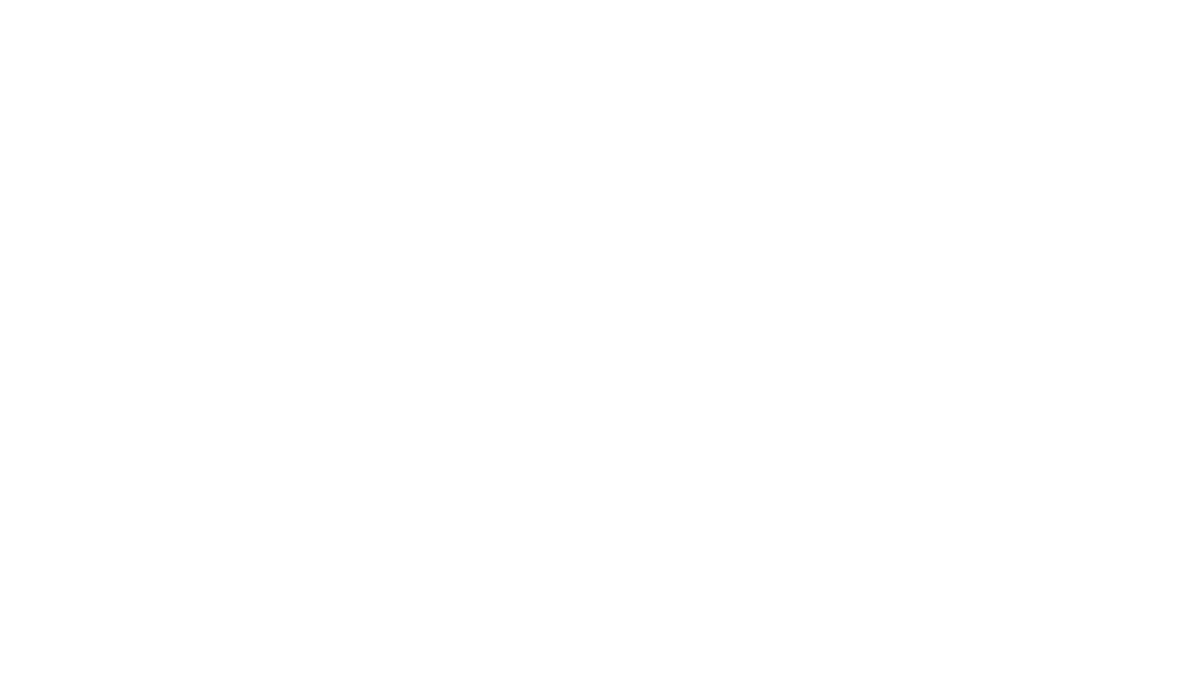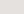Primož Rome

Saturday, January 15, 2022 · 16 min read

# Volts, Currents, and the Basic Concepts of Electricity

Electricity is the generated flow of electrons through pathways to power everyday objects like computers, heaters, and light bulbs.## Important electricity terms

• Conductors and Insulators: Good conductors are materials that let electricity flow through them easily. Most metals are good conductors, which is why metal is used in electrical wiring all over the world. The opposites of conductors are insulators. Insulators don't carry electricity well. We need insulators to protect people from flowing electricity. Rubber and plastics are examples of good insulators

• Voltage: Voltage is the force that makes electrons flow. It's a difference in potential energy between two different points in a circuit.

• Current: Current is the rate of the flow of electrons. It's measured in amperes, which are also called amps.

• Power (Watts): The power used in a circuit is measured in watts. Watts are calculated by multiplying the voltage by the current.

• Resistance: This is the measure of how well something conducts electricity. If it has a low resistance, the object is a great conductor of electricity, and if it has a high resistance, that means that it doesn't conduct electricity well.

• Other Important Electrical Terms

• Introduction to Electricity

• Electricity Fundamentals

## What is an electric current?

An electric current is a flow of electrons. Current flows through a circuit when there's a difference in potential energy between one end of a conductor and the other.

### Flow of electrons

In a complete circuit, the electrons flow from the negative terminal of the battery to the positive terminal. It looks like a positive charge is moving in the other direction, but actually, the positive particles stay still.

### How Is current measured?

Current is measured in amps, and this measurement is taken by calculating the amount of charge that flows through one point in a circuit. In electrical equations, a current is indicated using the letter "I."

### Calculating current

Current can be calculated using Ohm's Law. The equation for Ohm's Law is I = V/R, where I is the current, V is the voltage, and R is the resistance. This equation can also be used to figure out the resistance of a circuit or the voltage of a circuit if the other factors are known.

Current can also be used to calculate the power using the equation P = I * V, where P is power, I is current, and V is voltage.

### AC and DC

• Direct current (DC) is a constant flow of electrical charge in a single direction. The inner workings of most electronics use direct current

• Alternating current (AC) is a current in which the flow of the electrical charge constantly switches directions. Power lines in the United States transmit power using alternating currents.

## What is an electronic circuit?

Electronic circuits are pathways used to transmit electric currents and move electricity. Electronic circuits make it possible for electrical devices to work by drawing power from a battery or other power source.

## Parts of a circuit

There are three main parts of a circuit: the power source, wires to conduct the electricity, and a device at the other end to use the electricity. The power source can be a battery or a wall outlet connected to a power grid. The wires are made of metal because it's a good conductor, and they are wrapped in plastic to insulate the flowing electricity so it stays inside the wires. Circuits also usually contain switches, which allow you to temporarily break the circuit without dismantling it. This allows you to turn the device being powered on and off.

## Series circuit

A series circuit is a type of circuit where all of the parts are connected in a continuous line that forms a loop. Since every part is connected one after another, if one part stops working, the whole circuit stops working.

## Parallel circuit

A parallel circuit contains multiple pathways, so the electricity can be split and sent to different places at once. has different pathways in the circuit so the electricity is divided as it moves through the circuit. Some electricity will follow one path, while some of it follows another path. When you cut the power to part of a parallel circuit, the rest of the circuit keeps working.

## Series-parallel circuit

A series-parallel circuit contains both parallel-connected and series-connected parts. This type of circuit is trickier to work with because you need to understand which parts are connected in series and which are connected in parallel.

## Closed, open, and short circuits

A closed-circuit is a fully connected circuit that allows the electricity to flow through it uninterrupted. When a circuit is broken, it becomes an open circuit; the electricity stops flowing at the point where the connection was severed. The third type of circuit is the most dangerous: a short circuit. A short circuit is when electricity flows between two points that aren't supposed to be connected. This happens because electricity will always take the easiest path; if there's a "shortcut," it will flow that way.